#Want to know how Deep Learning works? Here’s a quick guide for everyone

Once you’ve read this article, you will understand the basics of AI and ML. More importantly, you will understand how Deep Learning, the most popular type of ML, works.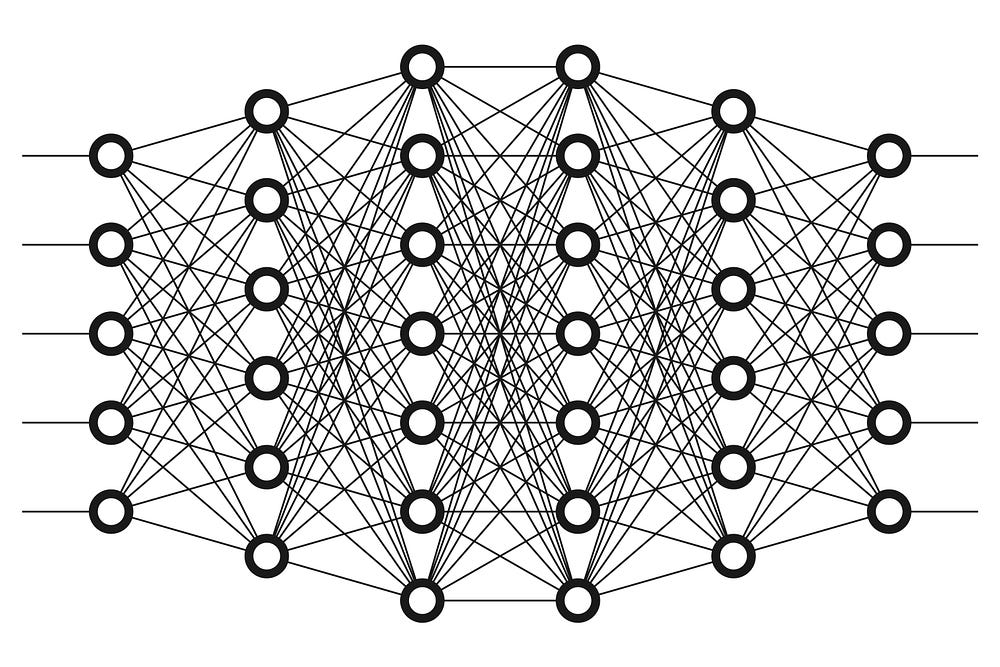Image credit: Datanami

Artificial Intelligence (AI) and Machine Learning (ML) are some of the hottest topics right now.

The term “AI” is thrown around casually every day. You hear aspiring developers saying they want to learn AI. You also hear executives saying they want to implement AI in their services. But quite often, many of these people don’t understand what AI is.

Once you’ve read this article, you will understand the basics of AI and ML. More importantly, you will understand how Deep Learning, the most popular type of ML, works.

This guide is intended for everyone, so no advanced mathematics will be involved.

### Background

The first step towards understanding how Deep Learning works is to grasp the differences between important terms.

Artificial Intelligence vs Machine Learning

Artificial Intelligence is the replication of human intelligence in computers.

When AI research first started, researchers were trying to replicate human intelligence for specific tasks — like playing a game.

They introduced a vast number of rules that the computer needed to respect. The computer had a specific list of possible actions, and made decisions based on those rules.

Machine Learning refers to the ability of a machine to learn using large data sets instead of hard coded rules.

ML allows computers to learn by themselves. This type of learning takes advantage of the processing power of modern computers, which can easily process large data sets.

Supervised learning vs unsupervised learning

Supervised Learning involves using labelled data sets that have inputs and expected outputs.

When you train an AI using supervised learning, you give it an input and tell it the expected output.

If the output generated by the AI is wrong, it will readjust its calculations. This process is done iteratively over the data set, until the AI makes no more mistakes.

An example of supervised learning is a weather-predicting AI. It learns to predict weather using historical data. That training data has inputs (pressure, humidity, wind speed) and outputs (temperature).

Unsupervised Learning is the task of machine learning using data sets with no specified structure.

When you train an AI using unsupervised learning, you let the AI make logical classifications of the data.

An example of unsupervised learning is a behavior-predicting AI for an e-commerce website. It won’t learn by using a labelled data set of inputs and outputs.

Instead, it will create its own classification of the input data. It will tell you which kind of users are most likely to buy different products.

### Now, how does Deep Learning work?

You’re now prepared to understand what Deep Learning is, and how it works.

Deep Learning is a machine learning method. It allows us to train an AI to predict outputs, given a set of inputs. Both supervised and unsupervised learning can be used to train the AI.

We will learn how deep learning works by building an hypothetical airplane ticket price estimation service. We will train it using a supervised learning method.

We want our airplane ticket price estimator to predict the price using the following inputs (we are excluding return tickets for simplicity):

• Origin Airport
• Destination Airport
• Departure Date
• Airline

Neural networks

Let’s look inside the brain of our AI.

Like animals, our estimator AI’s brain has neurons. They are represented by circles. These neurons are inter-connected.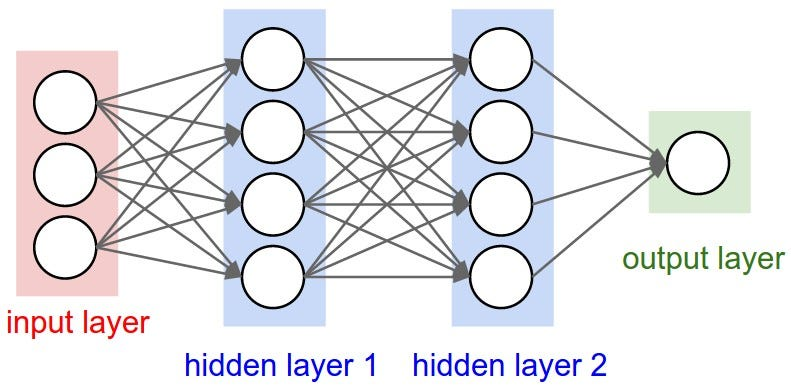Image credit: CS231n

The neurons are grouped into three different types of layers:

1. Input Layer
2. Hidden Layer(s)
3. Output Layer

The input layer receives input data. In our case, we have four neurons in the input layer: Origin Airport, Destination Airport, Departure Date, and Airline. The input layer passes the inputs to the first hidden layer.

The hidden layers perform mathematical computations on our inputs. One of the challenges in creating neural networks is deciding the number of hidden layers, as well as the number of neurons for each layer.

The “Deep” in Deep Learning refers to having more than one hidden layer.

The output layer returns the output data. In our case, it gives us the price prediction.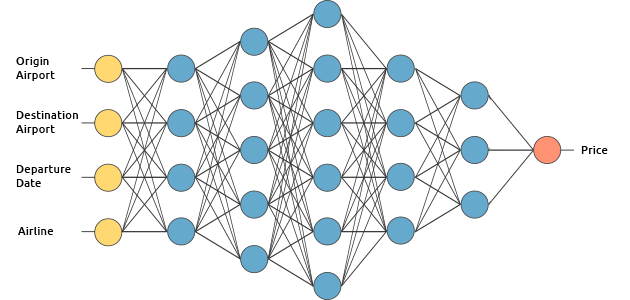So how does it compute the price prediction?

This is where the magic of Deep Learning begins.

Each connection between neurons is associated with a weight. This weight dictates the importance of the input value. The initial weights are set randomly.

When predicting the price of an airplane ticket, the departure date is one of the heavier factors. Hence, the departure date neuron connections will have a big weight.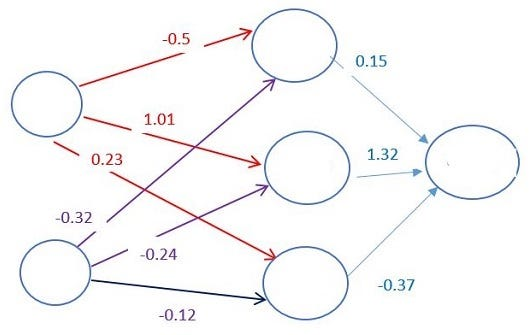Image credit: CodeProject

Each neuron has an Activation Function. These functions are hard to understand without mathematical reasoning.

Simply put, one of its purposes is to “standardize” the output from the neuron.

Once a set of input data has passed through all the layers of the neural network, it returns the output data through the output layer.

Nothing complicated, right?

Training the Neural Network

Training the AI is the hardest part of Deep Learning. Why?

1. You need a large data set.
2. You need a large amount of computational power.

For our airplane ticket price estimator, we need to find historical data of ticket prices. And due to the large amount of possible airports and departure date combinations, we need a very large list of ticket prices.

To train the AI, we need to give it the inputs from our data set, and compare its outputs with the outputs from the data set. Since the AI is still untrained, its outputs will be wrong.

Once we go through the whole data set, we can create a function that shows us how wrong the AI’s outputs were from the real outputs. This function is called the Cost Function.

Ideally, we want our cost function to be zero. That’s when our AI’s outputs are the same as the data set outputs.

How can we reduce the cost function?

We change the weights between neurons. We could randomly change them until our cost function is low, but that’s not very efficient.

Gradient Descent is a technique that allows us to find the minimum of a function. In our case, we are looking for the minimum of the cost function.

It works by changing the weights in small increments after each data set iteration. By computing the derivative (or gradient) of the cost function at a certain set of weight, we’re able to see in which direction the minimum is.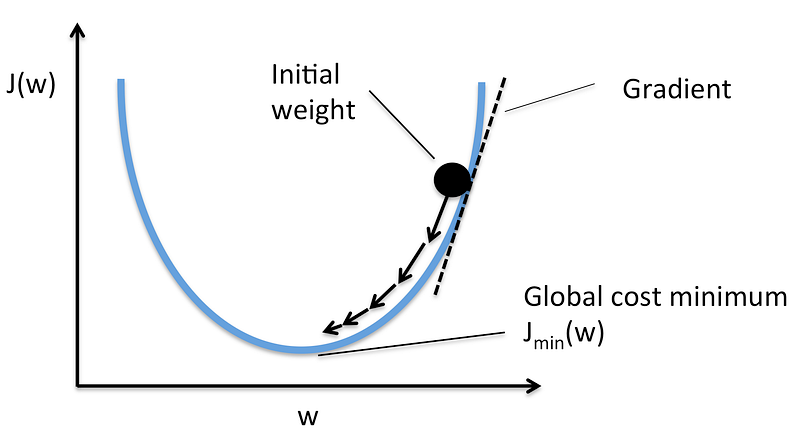Image credit: Sebastian Raschka

To minimize the cost function, you need to iterate through your data set many times. This is why you need a large amount of computational power.

Updating the weights using gradient descent is done automatically. That’s the magic of Deep Learning!

Once we’ve trained our airplane ticket price estimator AI, we can use it to predict future prices.

### Where can I learn more?

There are many other types of neural networks: Convolutional Neural Networks for Computer Vision and Recurrent Neural Networks for Natural Language Processing.

If you want to learn the technical aspect of Deep Learning, I suggest taking an online course.

Currently, one of the best courses for Deep Learning is Andrew Ng’s Deep Learning Specialization. If you’re not interested in getting a certificate, you don’t need to pay for the course. You can audit it for free instead.

If you have any questions, or want more technical explanations of the concepts, please ask below!

### In summary…

• Deep Learning uses a Neural Network to imitate animal intelligence.
• There are three types of layers of neurons in a neural network: the InputLayer, the Hidden Layer(s), and the Output Layer.
• Connections between neurons are associated with a weight, dictating the importance of the input value.
• Neurons apply an Activation Function on the data to “standardize” the output coming out of the neuron.
• To train a Neural Network, you need a large data set.
• Iterating through the data set and comparing the outputs will produce a Cost Function, indicating how much the AI is off from the real outputs.
• After every iteration through the data set, the weights between neurons are adjusted using Gradient Descent to reduce the cost function.

You can also check out my explanation of how I used Python to find interesting people to follow on Medium.

Thanks to Jonathan Sugumar.